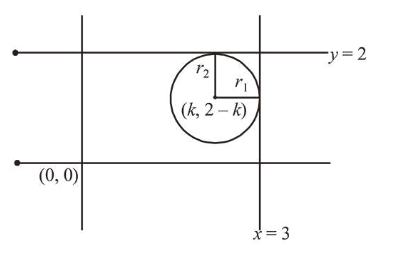# The diameter of the circle,Question:

The diameter of the circle, whose centre lies on the line $x+y=2$ in the first quadrant and which touches both the lines $x=3$ and $y=2$, is_______.

Solution:$\Rightarrow$ Radius $\left(r_{1}\right)=3-k$

$\because$ Centre lies on $x+y=2$

Let $x=k$

$\therefore y=2-k$

$\Rightarrow$ Centre $=(k, 2-k)$

Also, radius $\left(r_{2}\right)=2-(2-k)$

$\therefore 3-k=2-(2-k)$

$\Rightarrow k=\frac{3}{2}$

$r=3-\frac{3}{2}=\frac{3}{2}$

Hence, diameter $=3$.